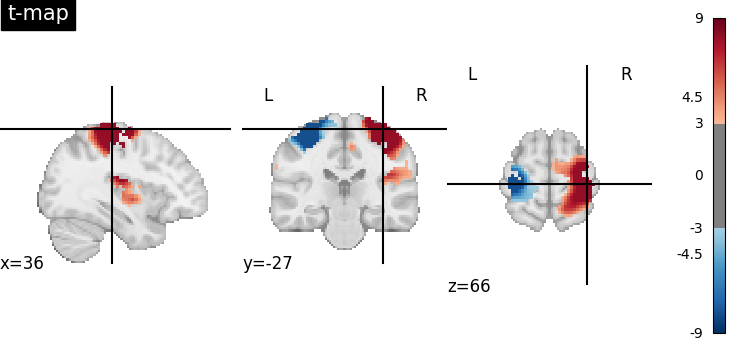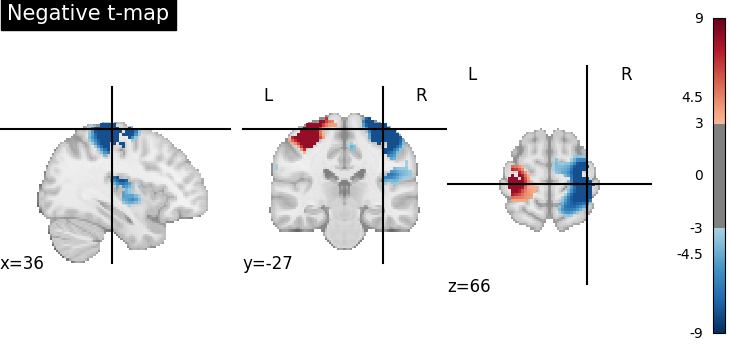# 9.7.1. Negating an image with math_img¶

The goal of this example is to illustrate the use of the function `nilearn.image.math_img` on T-maps. We compute a negative image by multiplying its voxel values with -1.

```from nilearn import datasets, plotting, image
```

Retrieve the data: the localizer dataset with contrast maps.

```motor_images = datasets.fetch_neurovault_motor_task()
stat_img = motor_images.images
```

Multiply voxel values by -1.

```negative_stat_img = image.math_img("-img", img=stat_img)

plotting.plot_stat_map(stat_img,
cut_coords=(36, -27, 66),
threshold=3, title="t-map", vmax=9
)
plotting.plot_stat_map(negative_stat_img,
cut_coords=(36, -27, 66),
threshold=3, title="Negative t-map", vmax=9
)
plotting.show()
```
••Total running time of the script: ( 0 minutes 5.882 seconds)

Estimated memory usage: 9 MB

Gallery generated by Sphinx-Gallery